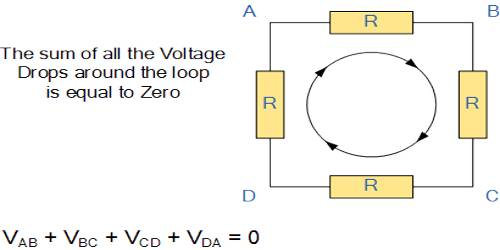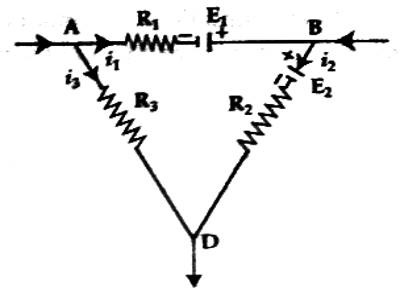# Kirchoff’s Second Law: The Voltage Law

Kirchoff’s Second Law: The Voltage Law

Second law: The total emf within a closed circuit is equal to the algebraic sum of the products of currents and resistances in different branches of that circuit.

Alternatively, in any network of conductors, if we consider a closed circuit, then the algebraic sum of the products of the current and resistance of each part of the circuit is equal to the total emf in that circuit i.e., ΣiR = ΣE.

This law is known as Kirchhoff’s voltage law.

Kirchhoff’s Voltage Law or KVL, states that “in any closed loop network, the total voltage around the loop is equal to the sum of all the voltage drops within the same loop” which is also equal to zero. In other words, the algebraic sum of all voltages within the loop must be equal to zero. This idea by Kirchhoff is known as the Conservation of Energy.

Explanation: In a closed circuit direction of current in some part can be anticlockwise and in some part, it can be clockwise. For this reason, the product of current and resistance in case of the clockwise flow of current is to be taken as positive. In this consideration, if any cell or battery sends current in the clockwise direction, it’s e.m.f. is to be considered positive, while the e.m.f. is to be considered negative if the cell or battery sends current in the anti-clockwise direction. In the figure, ABDA indicates a closed circuit. The resistances of parts AB. BD and DA are respectively R1, R2, and R3; currents in parts AB and BD respectively i1 and i2 are clockwise and current i3 in part AD is anti-clockwise.Besides, the electric cell of part AB having e.m.f. E1 tends to send current clockwise and electric cell of part BD having emf E2 tends to send current in anticlockwise. So, taking clockwise current as positive and anticlockwise current as negative Kirchhoff’s second law can be written as,

i1R1 + i2R2 – i3R3 = E1 – E2

or, i1R1 + i2R2 + (– i3R3) = E1 + (– E2)

Symbolically, the above equation can be written as, Σir = ΣE.

If there is no e.m.f. in the circuit, Σir = 0.

[N.B. For clockwise current, if the product of current and resistance is taken as negative, then for anticlockwise current that product is to be taken as positive. In this case, emf is to be denoted like this.]